# RD Sharma Solutions for Class 10 Chapter 6 Trigonometric Identities Exercise 6.2

Trigonometric ratios in terms of the value of one of them are majorly focused in this exercise. The RD Sharma Solutions Class 10 is a one-stop solution for students to develop good problem-solving skills in various chapters of RD Sharma. All the solutions are prepared by expert teams at BYJU’S following the CBSE patterns. Students can download RD Sharma Solutions for Class 10 Maths Chapter 6 Trigonometric Identities Exercise 6.2 PDF below for complete assistance.

## RD Sharma Solutions for Class 10 Chapter 6 Trigonometric Identities Exercise 6.2 Download PDF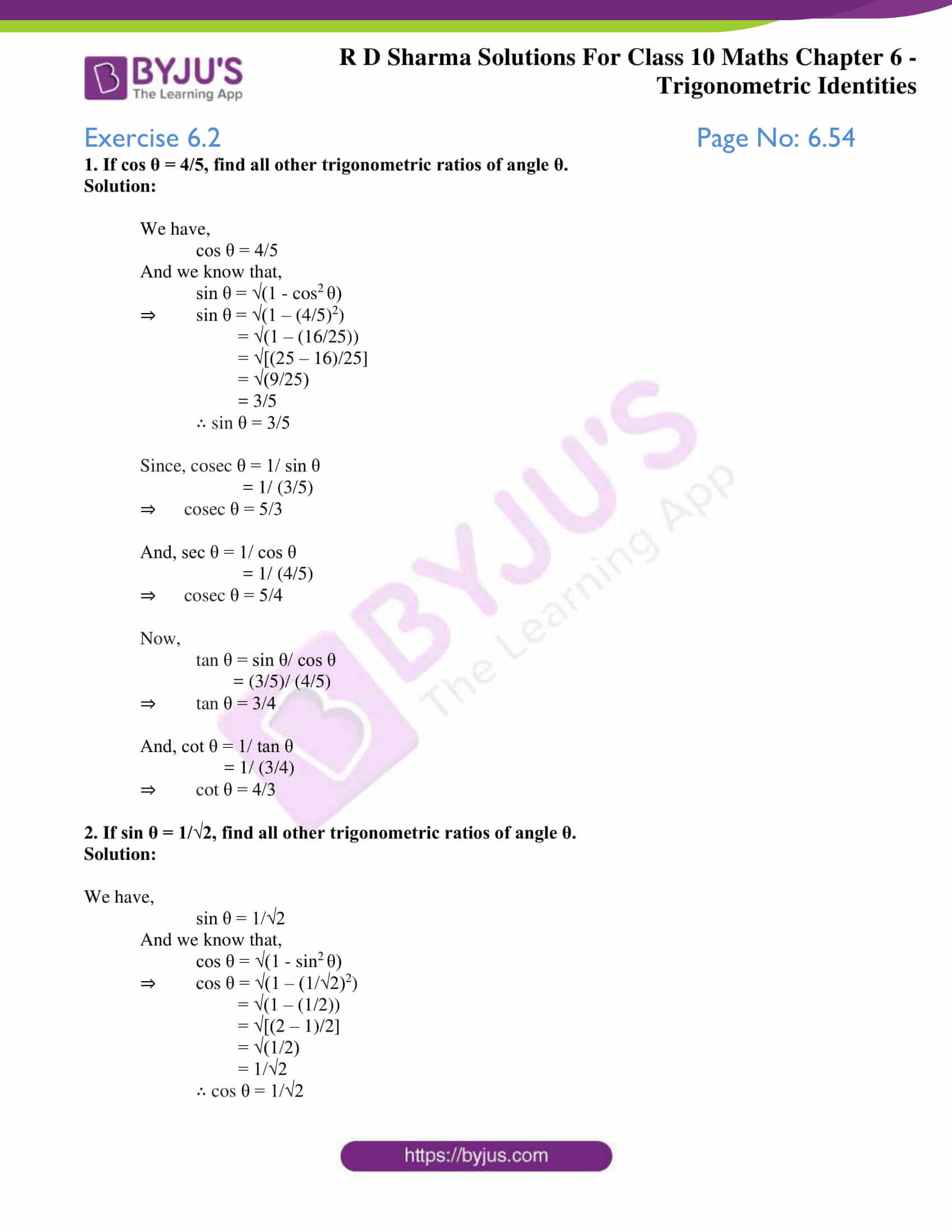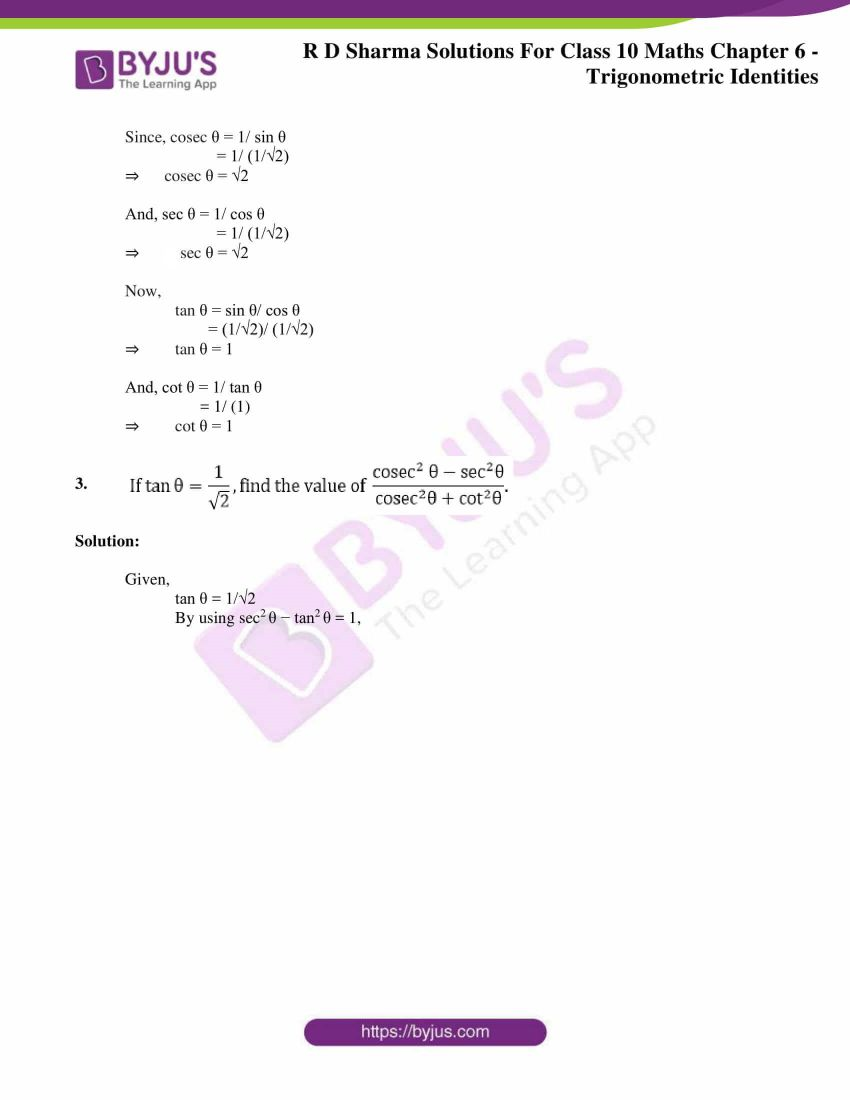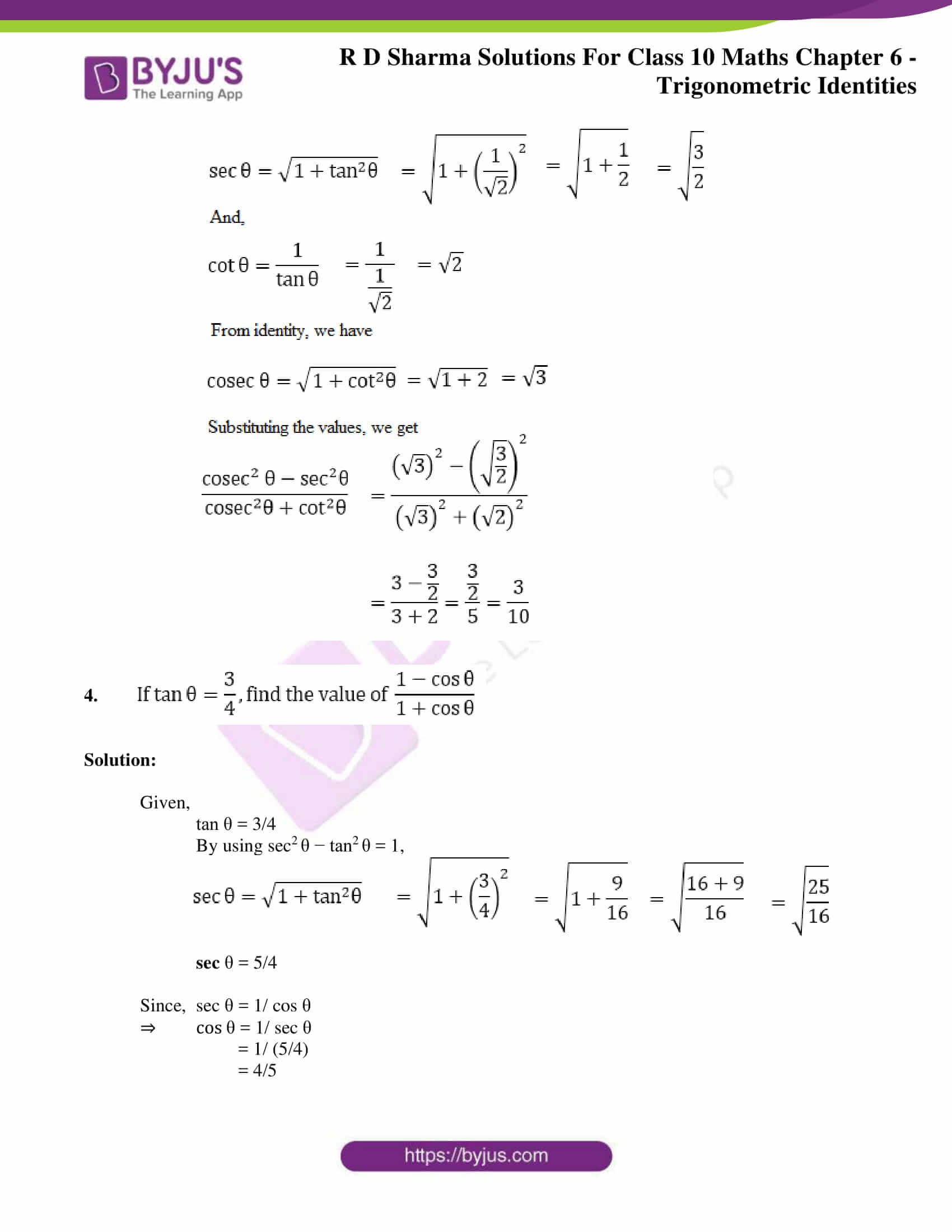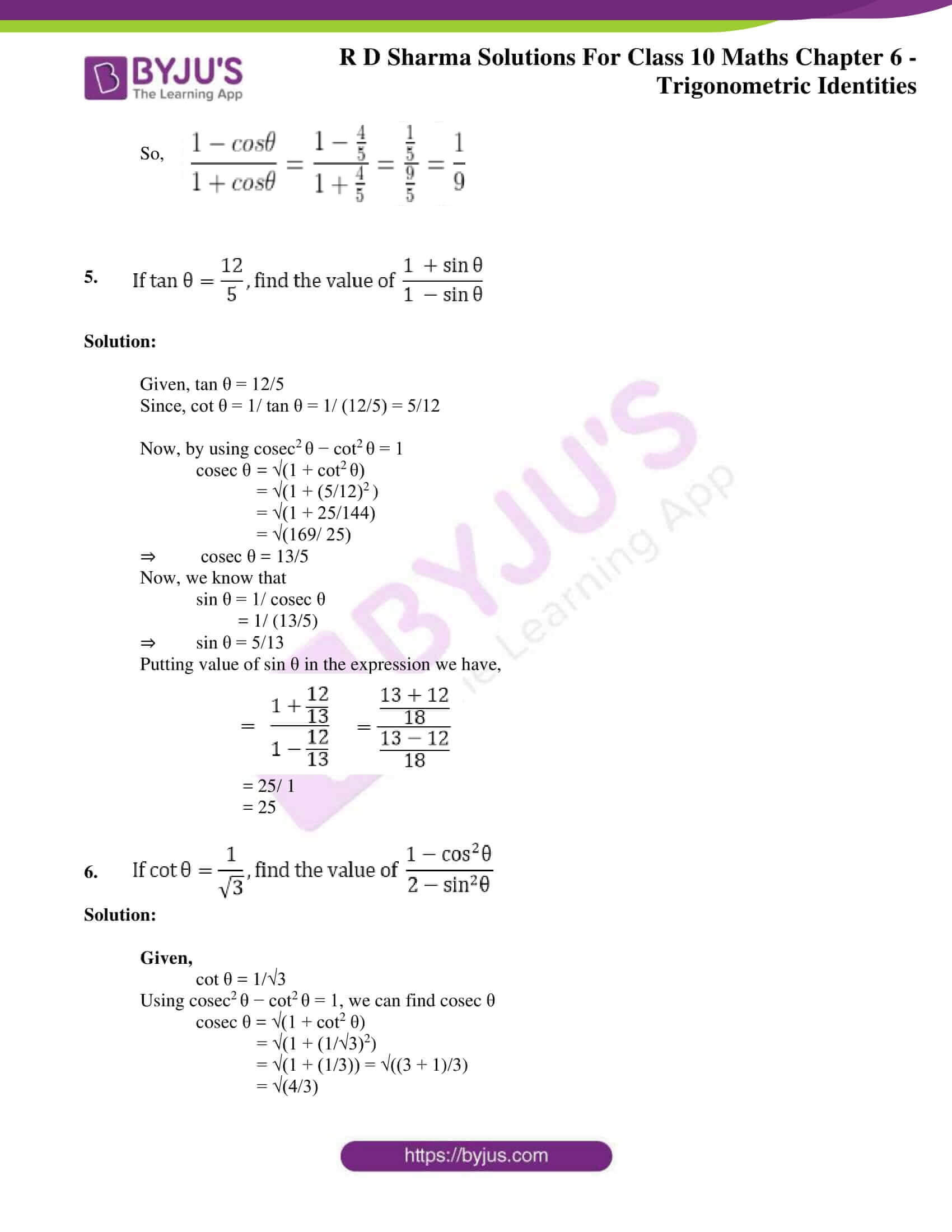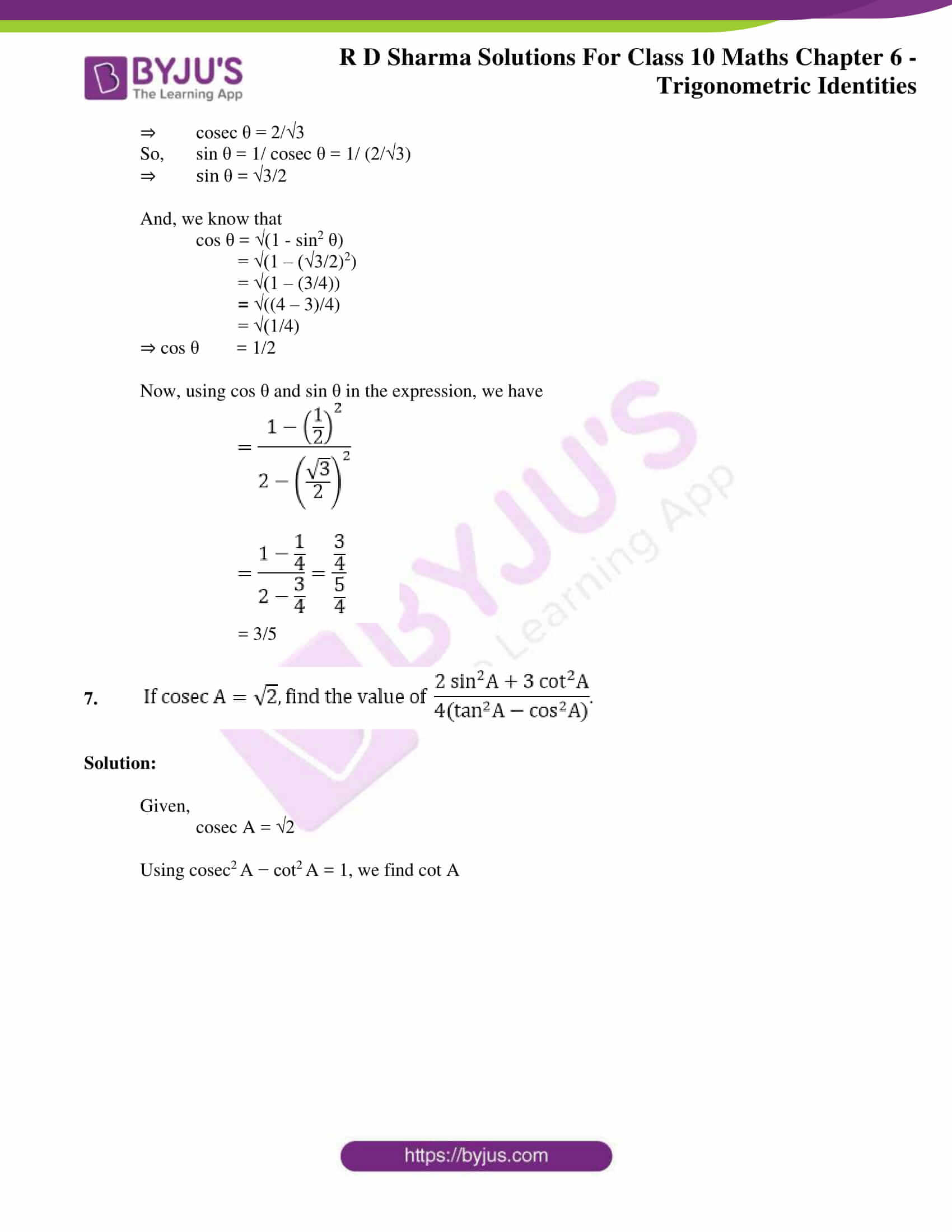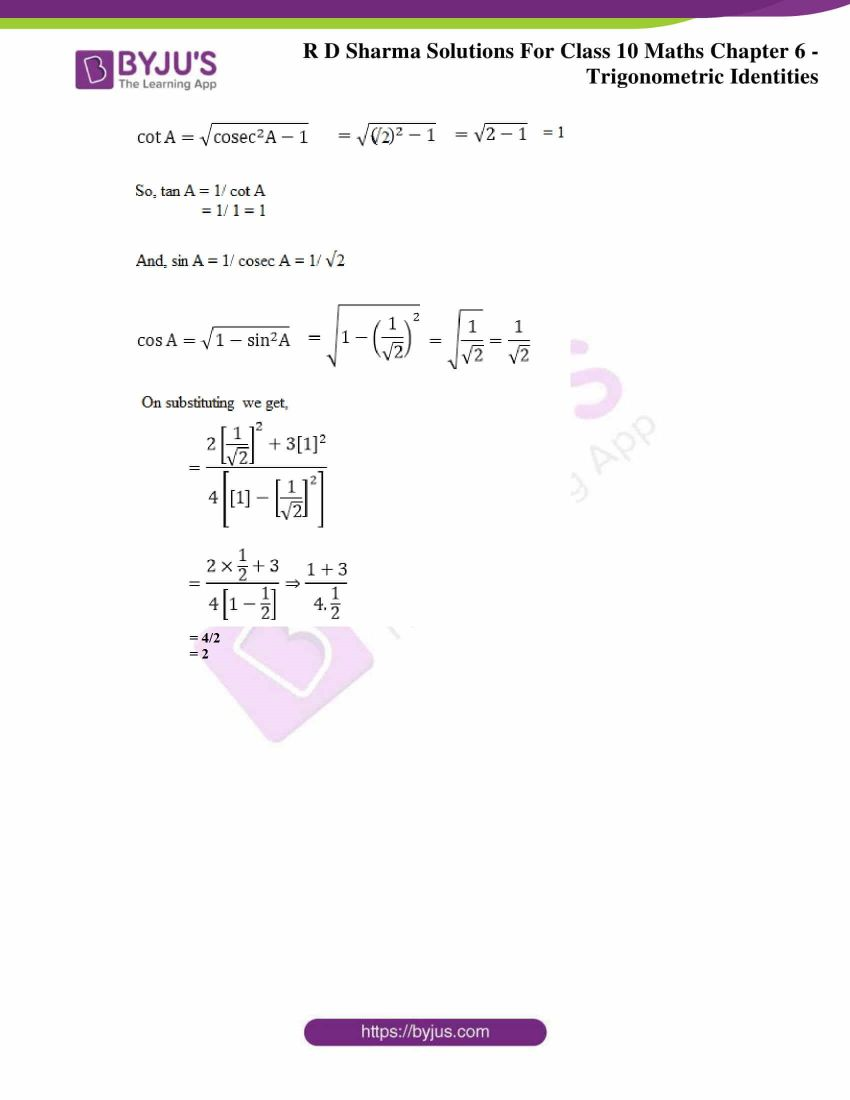### Access RD Sharma Solutions for Class 10 Chapter 6 Trigonometric Identities Exercise 6.2

1. If cos θ = 4/5, find all other trigonometric ratios of angle θ.

Solution:

We have,

cos θ = 4/5

And we know that,

sin θ = √(1 – cos2 θ)

⇒ sin θ = √(1 – (4/5)2)

= √(1 – (16/25))

= √[(25 – 16)/25]

= √(9/25)

= 3/5

∴ sin θ = 3/5

Since, cosec θ = 1/ sin θ

= 1/ (3/5)

⇒ cosec θ = 5/3

And, sec θ = 1/ cos θ

= 1/ (4/5)

⇒ cosec θ = 5/4

Now,

tan θ = sin θ/ cos θ

= (3/5)/ (4/5)

⇒ tan θ = 3/4

And, cot θ = 1/ tan θ

= 1/ (3/4)

⇒ cot θ = 4/3

2. If sin θ = 1/√2, find all other trigonometric ratios of angle θ.

Solution:

We have,

sin θ = 1/√2

And we know that,

cos θ = √(1 – sin2 θ)

⇒ cos θ = √(1 – (1/√2)2)

= √(1 – (1/2))

= √[(2 – 1)/2]

= √(1/2)

= 1/√2

∴ cos θ = 1/√2

Since, cosec θ = 1/ sin θ

= 1/ (1/√2)

⇒ cosec θ = √2

And, sec θ = 1/ cos θ

= 1/ (1/√2)

⇒ sec θ = √2

Now,

tan θ = sin θ/ cos θ

= (1/√2)/ (1/√2)

⇒ tan θ = 1

And, cot θ = 1/ tan θ

= 1/ (1)

⇒ cot θ = 1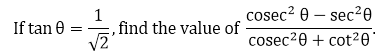3.

Solution:

Given,

tan θ = 1/√2

By using sec2 θ − tan2 θ = 1,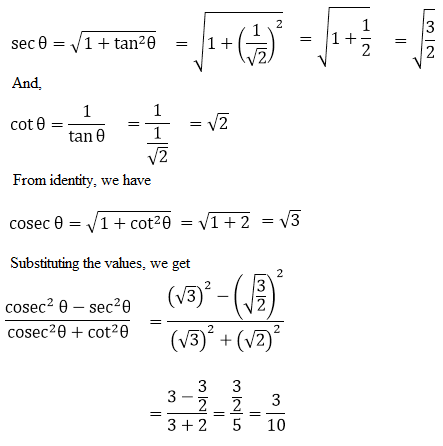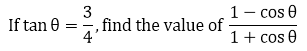4.

Solution:

Given,

tan θ = 3/4

By using sec2 θ − tan2 θ = 1,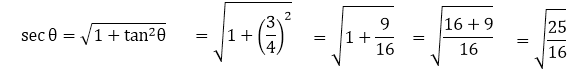sec θ = 5/4

Since, sec θ = 1/ cos θ

⇒ cos θ = 1/ sec θ

= 1/ (5/4)

= 4/5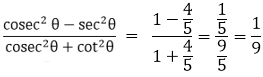So,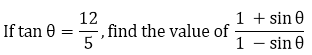5.

Solution:

Given, tan θ = 12/5

Since, cot θ = 1/ tan θ = 1/ (12/5) = 5/12

Now, by using cosec2 θ − cot2 θ = 1

cosec θ = √(1 + cot2 θ)

= √(1 + (5/12)2 )

= √(1 + 25/144)

= √(169/ 25)

⇒ cosec θ = 13/5

Now, we know that

sin θ = 1/ cosec θ

= 1/ (13/5)

⇒ sin θ = 5/13

Putting value of sin θ in the expression we have,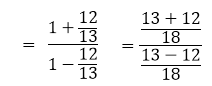= 25/ 1

= 25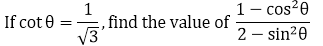6.

Solution:

Given,

cot θ = 1/√3

Using cosec2 θ − cot2 θ = 1, we can find cosec θ

cosec θ = √(1 + cot2 θ)

= √(1 + (1/√3)2)

= √(1 + (1/3)) = √((3 + 1)/3)

= √(4/3)

⇒ cosec θ = 2/√3

So, sin θ = 1/ cosec θ = 1/ (2/√3)

⇒ sin θ = √3/2

And, we know that

cos θ = √(1 – sin2 θ)

= √(1 – (√3/2)2)

= √(1 – (3/4))

= √((4 – 3)/4)

= √(1/4)

⇒ cos θ = 1/2

Now, using cos θ and sin θ in the expression, we have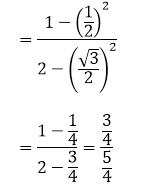= 3/5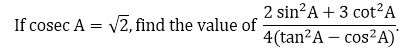7.

Solution:

Given,

cosec A = √2

Using cosec2 A − cot2 A = 1, we find cot A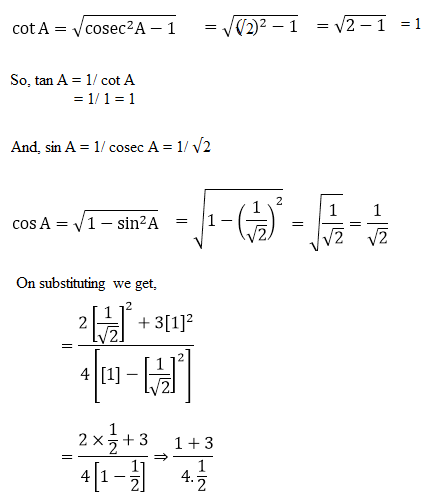= 4/2

= 2